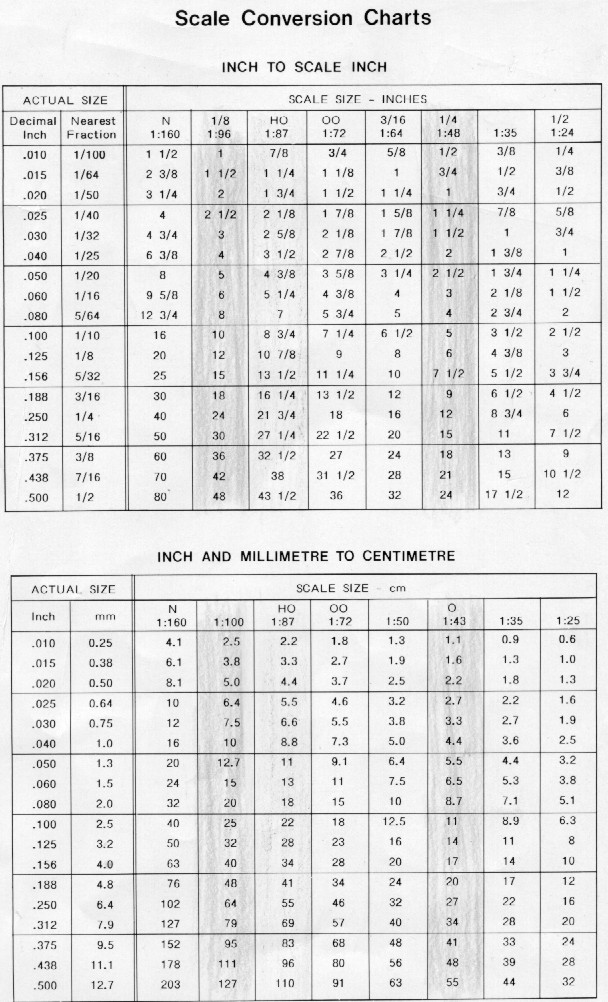[ Home ] [ What's New ] [ Newbies ] [ Scale Charts ] [ Towboat Info ] [ River Talk ] [ Construction101 ] [ Links & Kinks ] [ Miscellaneous ] [ Pilothouse ] [ On Deck ] [ Lighting ] [ Hulls ] [ Shafts ] [ Models ] [ Model Photography ] [ Plans ] [ Furr trip ] [ American Viking ] [ Vac-u-Boat ] [ Vac-U-Tow2 ] [ Detailing Menu ]
 Not sure what scale to build in? This chart should help in deciding a scale to build in. 1/2"=1' or 1:24 3/8"=1' or 1:32 1/4"=1' or 1:48 1/8"=1' or 1:96 298' BARGE 12' 5" 9' 3 3/4" 6' 2 1/2" 3' 1 1/4" 195' BARGE 8' 1 1/2" 6' 1 1/8" 4' 3/4" 2' 3/8" 115' BARGE 4' 9 1/2" 3' 7 1/8" 2' 4 3/4" 1' 2 3/8" 200' TOWBOAT 8' 4" 6' 3" 4' 2" 2' 1" 190' TOWBOAT 7' 11" 5' 9 3/4" 3' 10 1/2" 1' 7 3/4" 180' TOWBOAT 7' 6" 5' 7 1/2" 3' 9" 1' 10 1/2" 170' TOWBOAT 7' 1" 5' 3 3/4" 3' 6 1/2" 1' 9 1/4" 160' TOWBOAT 6' 10" 5' 0" 3' 4" 1' 8" 150' TOWBOAT 6' 3" 4' 8 1/2" 3' 1 1/2" 1' 6 3/4" 140' TOWBOAT 5' 10" 4' 4 1/2" 2' 11" 1' 5 1/2" 130' TOWBOAT 5' 5" 4' 3/4" 2' 8 1/2" 1' 4 1/4" 120' TOWBOAT 5' 0" 3' 9" 2' 6" 1' 8" 110' TOWBOAT 4' 7" 3' 5 1/4" 2' 3 1/2" 1' 1 3/4" 100' TOWBOAT 4' 2" 3' 1 1/2" 2' 1" 1' 1/2" 90' TOWBOAT 3' 9" 2' 9 3/4" 1' 10 1/2" 11 1/4" 80' TOWBOAT 3' 4" 2' 6" 1' 8" 10" 70' TOWBOAT 2' 11" 2' 2 1/4" 1' 5 1/2" 7 3/4" 60' TOWBOAT 2' 6" 1'7 1/2" 1' 3" 7 1/2" 55' TOWBOAT 2' 3 1/2" 1' 6 7/8" 1' 1 1/4" 6 7/8" 160' TOWBOAT with three 298' BARGES 43' 11" 32' 10 3/4" 21' 11 1/2" 10' 11 1/4" 160' TOWBOAT with five 195' BARGES 47 '3 1/2" 35' 4 3/4" 23' 7 3/4" 11' 9 7/8" 55' TOWBOAT with a 115' BARGE 7' 1" 5' 3 3/4" 3' 6 1/2" 1' 9 1/4"   Here's a scale I use for figures to decide if they'll fit my scale. If you measure in mm it is easier to tell what figure will work 1:24 1:32 1:48 1:96 14mm = 1' 10mm = 1' 7mm = 1' 3mm = 1' 84mm* 60mm* 42mm* 18mm* * Based on a 6' tall figure so keep in mind some people are shorter especially the women. There could also be a tall guy or two over 6' tall. Need to convert metric to standard or visa versa click link below http://www.worldwidemetric.com/metcal.htm DECIMAL EQUIVALENTS Here are decimal equivalents to fractions. Read down first column, then second, then third. 1/64 = .015625 23/64 = .359375 45/64 = .703125 1/32 = .03125 3/8 = .375 23/32 = .71875 3/64 = .046875 25/64 = .359375 47/64 = .734375 1/16 = .0625 13/32 = .40625 3/4 = .750 5/64 = .078125 27/64 = .421875 49/64 = .765625 3/32 = .09375 7/16 = .4375 25/32 = .78125 7/64 = .109375 29/64 = .453125 51/64 = .796875 1/8 = .125 15/32 = .46875 13/16 = .8125 9/64 = .140625 31/64 = .484375 53/64 = .828125 5/32 = .15625 1/2 = .500 27/32 = .84375 11/64 = .171875 33/64 = .515625 55/64 = .859375 3/16 = .1875 17/32 = .53125 7/8 = .875 13/64 = .203125 35/64 = .546875 57/64 = .890625 7/32 = .21875 9/16 = .5625 29/32 = .90625 15/64 = .234375 37/64 = .578125 59/64 = .921875 1/4 = .250 19/32 = .59375 15/16 =.9375 17/64 = .265625 39/64 = .609375 61/64=.953125 9/32 = .28125 5/8 = .625 31/32 =.96875 19/64 = .296875 41/64 = .640625 63/64 =.984375 5/16 = .3125 21/32 = .65625 1 = .1.000 21/64 = .328125 43/64 = .671875 11/32 = .34375 11/16 = .6875TM